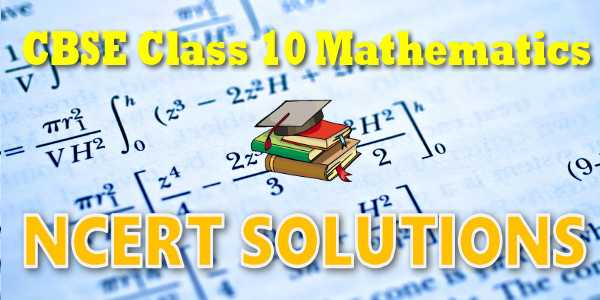No products in the cart.

# NCERT solutions for class 10 Mathematics## CBSE, JEE, NEET, NDA

Question Bank, Mock Tests, Exam Papers

NCERT Solutions, Sample Papers, Notes, Videos

## NCERT solutions for class 10 Mathematics## myCBSEguide App

Complete Guide for CBSE Students

NCERT Solutions, NCERT Exemplars, Revison Notes, Free Videos, CBSE Papers, MCQ Tests & more.## NCERT Solutions Chapter 7 Coordinate Geometry

In Class IX, you have studied that to locate the position of a point on a plane, we require a pair of coordinate axes. The distance of a point from the y-axis is called its x-coordinate, or abscissa. The distance of a point from the x-axis is called its y-coordinate, or ordinate. The coordinates of a point on the x-axis are of the form (x, 0), and of a point on the y-axis are of the form (0, y). Here is a play for you. Draw a set of a pair of perpendicular axes on a graph paper. Now plot the following points and join them as directed: Join the point A(4, 8) to B(3, 9) to C(3, 8) to D(1, 6) to E(1, 5) to F(3, 3) to G(6, 3) to H(8, 5) to I(8, 6) to J(6, 8) to K(6, 9) to L(5, 8) to A. Then join the points P(3.5, 7), Q (3, 6) and R(4, 6) to form a triangle. Also join the points X(5.5, 7), Y(5, 6) and Z(6, 6) to form a triangle. Now join S(4, 5), T(4.5, 4) and U(5, 5) to form a triangle. Lastly join S to the points (0, 5) and (0, 6) and join U to the points (9, 5) and (9, 6). What picture have you got? Also, you have seen that a linear equation in two variables of the form ax + by + c = 0, (a, b are not simultaneously zero), when represented graphically, gives a straight line. Further, in Chapter 2, you have seen the graph of y = ax2 + bx + c (a ≠ 0), is a parabola. In fact, coordinate geometry has been developed as an algebraic tool for studying geometry of figures. It helps us to study geometry using algebra, and understand algebra with the help of geometry. Because of this,
coordinate geometry is widely applied in various fields such as physics, engineering, navigation, seismology and art! In this chapter, you will learn how to find the distance between the two points whose coordinates are given, and to find the area of the triangle formed by three given points. You will also study how to find the coordinates of the point which divides a line segment joining two given points in a given ratio

## NCERT Solutions for Class 10th Mathematics

NCERT Solutions Class 10 Mathematics PDF (Download) Free from myCBSEguide app and myCBSEguide website. Ncert solution class 10 Mathematics includes text book solutions from both part 1. NCERT Solutions for CBSE Class 10 Mathematics have total 15 chapters. Class 10 Mathematics ncert Solutions in pdf for free Download are given in this website. Ncert Mathematics class 10 solutions PDF and Mathematics ncert class 10 PDF solutions with latest modifications and as per the latest CBSE syllabus are only available in myCBSEguide.

## NCERT Solutions for Class 10## myCBSEguide

Trusted by 1 Crore+ Students

#### Question Paper Creator

• Create papers in minutes
• Print with your name & Logo